The OpenFOAM FoundationVectorSpace< Form, Cmpt, Ncmpts > Class Template Reference

Templated vector space. More...

Inheritance diagram for VectorSpace< Form, Cmpt, Ncmpts >: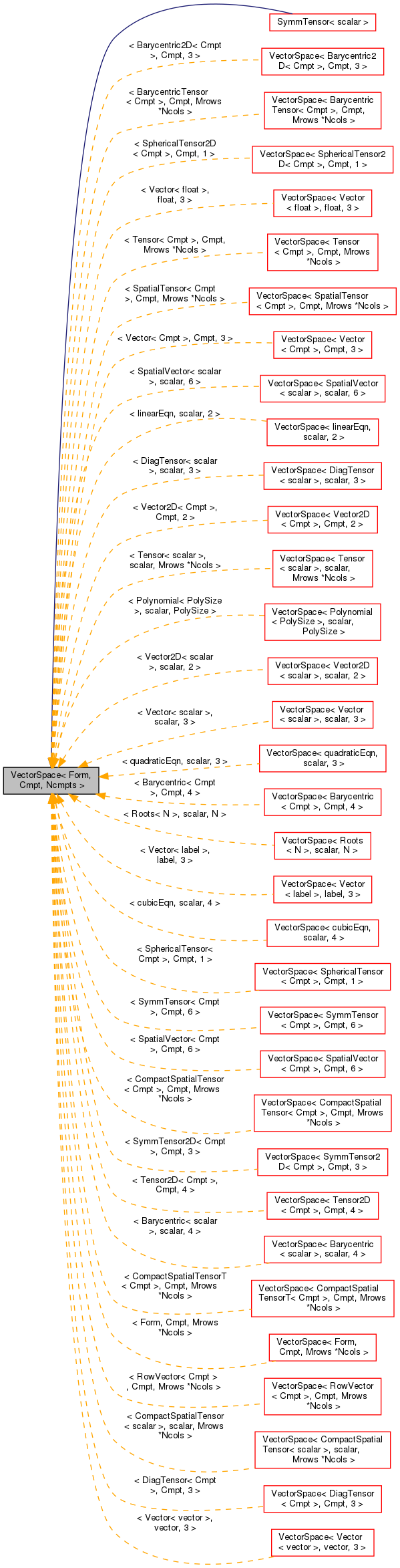[legend]
Collaboration diagram for VectorSpace< Form, Cmpt, Ncmpts >: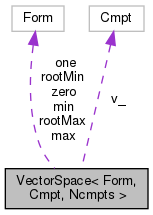[legend]

## Classes

class  ConstBlock
Const sub-block type. More...

## Public Types

typedef VectorSpace< Form, Cmpt, Ncmpts > vsType
VectorSpace type. More...

typedef Cmpt cmptType
Component type. More...

## Public Member Functions

VectorSpace ()
Construct null. More...

VectorSpace (const Foam::zero)
Construct initialized to zero. More...

VectorSpace (Istream &)
Construct from Istream. More...

template<class Form2 , class Cmpt2 >
VectorSpace (const VectorSpace< Form2, Cmpt2, Ncmpts > &)
Construct as copy of a VectorSpace with the same size. More...

const Cmpt & component (const direction) const

Cmpt & component (const direction)

void component (Cmpt &, const direction) const

void replace (const direction, const Cmpt &)

template<class SubVector , direction BStart>
const ConstBlock< SubVector, BStart > block () const

const Cmpt & operator[] (const direction) const

Cmpt & operator[] (const direction)

void operator+= (const VectorSpace< Form, Cmpt, Ncmpts > &)

void operator-= (const VectorSpace< Form, Cmpt, Ncmpts > &)

void operator= (const Foam::zero)

void operator*= (const scalar)

void operator/= (const scalar)

template<class SubVector , direction BStart>
const VectorSpace< Form, Cmpt, Ncmpts >::template ConstBlock< SubVector, BStart > block () const

## Static Public Member Functions

static direction size ()
Return the number of elements in the VectorSpace = Ncmpts. More...

static Form uniform (const Cmpt &s)
Return a VectorSpace with all elements = s. More...

## Public Attributes

Cmpt v_ [Ncmpts]
The components of this vector space. More...

## Static Public Attributes

static const direction dim = 3
Dimensionality of space. More...

static const direction nComponents = Ncmpts
Number of components in this vector space. More...

static const direction mRows = Ncmpts

static const direction nCols = 1

static const char *const typeName = "symmTensor"

static const char *const componentNames []

static const Form zero

static const Form one

static const Form max

static const Form min

static const Form rootMax

static const Form rootMin

## Friends

Istreamoperator>> (Istream &, VectorSpace< Form, Cmpt, Ncmpts > &)

Ostreamoperator (Ostream &, const VectorSpace< Form, Cmpt, Ncmpts > &)

## Detailed Description

### template<class Form, class Cmpt, direction Ncmpts> class Foam::VectorSpace< Form, Cmpt, Ncmpts >

Templated vector space.

Template arguments are the Form the vector space will be used to create, the type of the elements and the number of elements.

Source files

Definition at line 53 of file VectorSpace.H.

## ◆ vsType

 typedef VectorSpace vsType

VectorSpace type.

Definition at line 87 of file VectorSpace.H.

## ◆ cmptType

 typedef Cmpt cmptType

Component type.

Definition at line 90 of file VectorSpace.H.

## ◆ VectorSpace() [1/4]

 VectorSpace ( )
inline

Construct null.

Definition at line 40 of file VectorSpaceI.H.

Here is the caller graph for this function: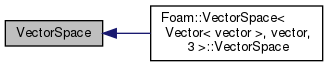## ◆ VectorSpace() [2/4]

 VectorSpace ( const Foam::zero )
inline

Construct initialized to zero.

Definition at line 45 of file VectorSpaceI.H.

## ◆ VectorSpace() [3/4]

 VectorSpace ( Istream & is )

Construct from Istream.

Definition at line 35 of file VectorSpace.C.

## ◆ VectorSpace() [4/4]

 VectorSpace ( const VectorSpace< Form2, Cmpt2, Ncmpts > & vs )
inlineexplicit

Construct as copy of a VectorSpace with the same size.

Definition at line 54 of file VectorSpaceI.H.

## ◆ size()

 direction size ( )
inlinestatic

Return the number of elements in the VectorSpace = Ncmpts.

Definition at line 83 of file VectorSpaceI.H.

Referenced by edgeCollapser::checkMeshQuality(), and createShellMesh::setRefinement().

Here is the caller graph for this function: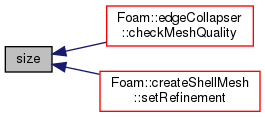## ◆ component() [1/3]

 const Cmpt & component ( const direction d ) const
inline

Definition at line 91 of file VectorSpaceI.H.

Here is the caller graph for this function: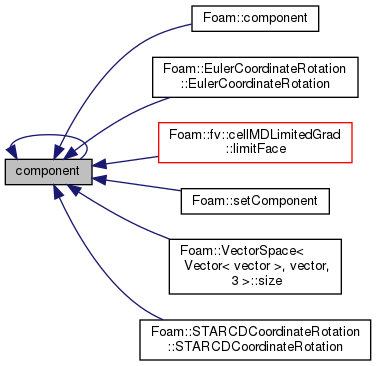## ◆ component() [2/3]

 Cmpt & component ( const direction d )
inline

Definition at line 110 of file VectorSpaceI.H.

## ◆ component() [3/3]

 void component ( Cmpt & c, const direction d ) const
inline

Definition at line 129 of file VectorSpaceI.H.

## ◆ replace()

 void replace ( const direction d, const Cmpt & c )
inline

Definition at line 149 of file VectorSpaceI.H.

Here is the caller graph for this function: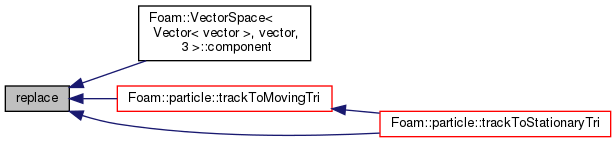## ◆ uniform()

 Form uniform ( const Cmpt & s )
inlinestatic

Return a VectorSpace with all elements = s.

Definition at line 168 of file VectorSpaceI.H.

## ◆ block() [1/2]

 const ConstBlock block ( ) const
inline

## ◆ operator[]() [1/2]

 const Cmpt & operator[] ( const direction d ) const
inline

Definition at line 190 of file VectorSpaceI.H.

## ◆ operator[]() [2/2]

 Cmpt & operator[] ( const direction d )
inline

Definition at line 209 of file VectorSpaceI.H.

## ◆ operator+=()

 void operator+= ( const VectorSpace< Form, Cmpt, Ncmpts > & vs )
inline

Definition at line 280 of file VectorSpaceI.H.

## ◆ operator-=()

 void operator-= ( const VectorSpace< Form, Cmpt, Ncmpts > & vs )
inline

Definition at line 290 of file VectorSpaceI.H.

## ◆ operator=()

 void operator= ( const Foam::zero )
inline

Definition at line 299 of file VectorSpaceI.H.

Here is the caller graph for this function: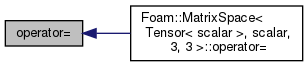## ◆ operator*=()

 void operator*= ( const scalar s )
inline

Definition at line 307 of file VectorSpaceI.H.

## ◆ operator/=()

 void operator/= ( const scalar s )
inline

Definition at line 317 of file VectorSpaceI.H.

## ◆ block() [2/2]

 const VectorSpace::template ConstBlock block ( ) const
inline

Definition at line 180 of file VectorSpaceI.H.

## ◆ operator>>

 Istream& operator>> ( Istream & , VectorSpace< Form, Cmpt, Ncmpts > & )
friend

## ◆ operator

 Ostream& operator ( Ostream & , const VectorSpace< Form, Cmpt, Ncmpts > & )
friend

## ◆ v_

 Cmpt v_[Ncmpts]

The components of this vector space.

Definition at line 84 of file VectorSpace.H.

## ◆ dim

 const direction dim = 3
static

Dimensionality of space.

Definition at line 96 of file VectorSpace.H.

Referenced by Foam::cof(), and Foam::det().

## ◆ nComponents

 const direction nComponents = Ncmpts
static

Number of components in this vector space.

Definition at line 99 of file VectorSpace.H.

Referenced by anisotropicFilter::operator()().

## ◆ mRows

 const direction mRows = Ncmpts
static

Definition at line 105 of file VectorSpace.H.

## ◆ nCols

 const direction nCols = 1
static

Definition at line 106 of file VectorSpace.H.

## ◆ typeName

 const char *const typeName = "symmTensor"
static

Definition at line 111 of file VectorSpace.H.

## ◆ componentNames

 const char *const componentNames
static
Initial value:
=
{
"xx", "xy", "xz",
"yy", "yz",
"zz"
}

Definition at line 112 of file VectorSpace.H.

## ◆ zero

 const Foam::symmTensor zero
static

Definition at line 113 of file VectorSpace.H.

## ◆ one

 const Foam::symmTensor one
static

Definition at line 114 of file VectorSpace.H.

## ◆ max

 const Foam::symmTensor max
static

## ◆ min

 const Foam::symmTensor min
static

## ◆ rootMax

 const Foam::symmTensor rootMax
static

Definition at line 117 of file VectorSpace.H.

## ◆ rootMin

 const Foam::symmTensor rootMin
static

Definition at line 118 of file VectorSpace.H.

The documentation for this class was generated from the following files: